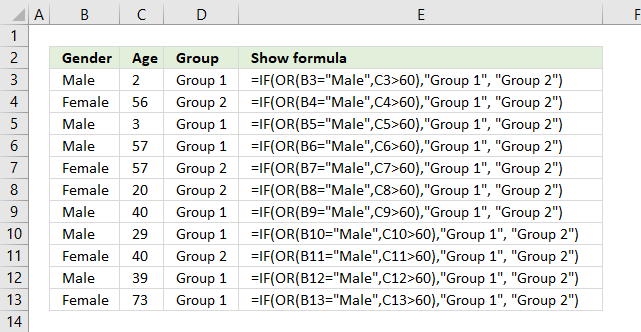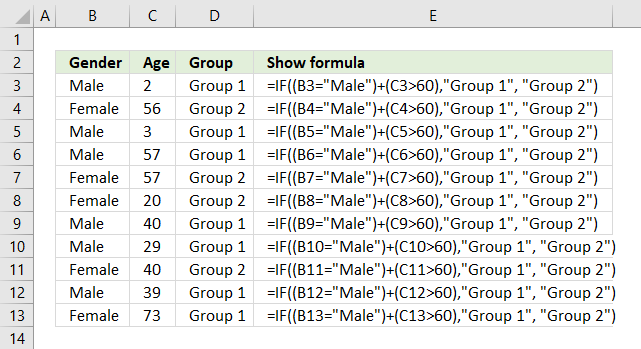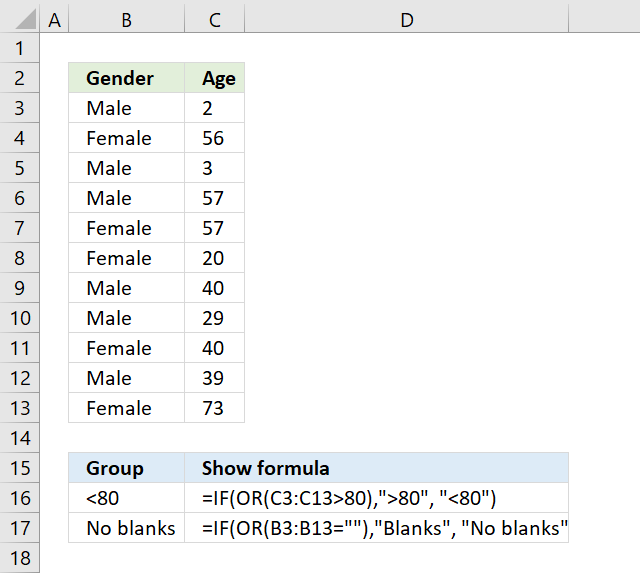Author: Oscar Cronquist Article last updated on November 30, 2017The formula above in cell D3 performs two different logical tests, if at least one of them is TRUE one thing happens, if all return FALSE another thing happens.

=IF(OR(B3="Male",C3>60),"Group 1", "Group 2")

If a value in column B is equal to "Male" or a value on the same row in column C is above 60 the formula returns "Group 1", if none of these logical expressions return TRUE the formula returns "Group 2"

### Alternative formulaYou can also wrap each logical expression with parentheses and use the + (plus sign) to have the same functionality as the OR function.

=IF((B3="Male")+(C3>60),"Group 1", "Group 2")

Why wrap each logical expression? It determines the order of calculation, you want the comparisons to be calculated before you add the boolean values.

You now know what a plus sign means if you happen to see a formula with a plus sign. The advantage is a slightly smaller formula.The array formula in cell B16 checks if there is a value larger than 80 in cell range C3:C13. You need to enter this as an array formula because you carry out a logical test to each cell in cell range C3:C13 and that logical test returns an array of boolean values.

=IF(OR(C3:C13>80),">80", "<80")

The OR function then returns TRUE if at least one boolean value is TRUE in the array or else FALSE. The IF function returns <80 if there are no values above 80 and >80 if there is at least one value above 80 in cell range C3:C13.

The array formula in cell B17 checks if there are any blank values in cell range B3:B13.

=IF(OR(B3:B13=""),"Blanks", "No blanks")

To enter an array formula press and hold CTRL + SHIFT simultaneously, then press Enter once. Release all keys.

The formula bar now shows the formula enclosed with curly brackets telling you that you entered the formula successfully. Don't enter the curly brackets yourself.